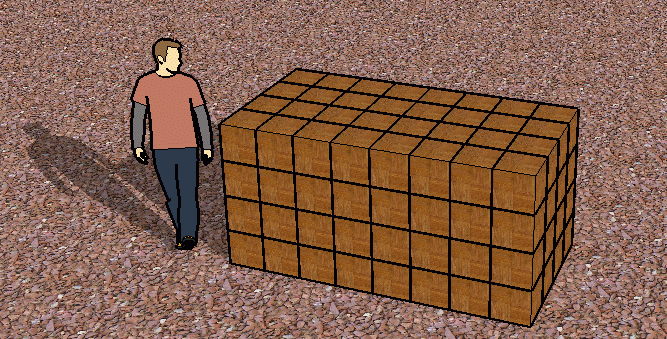SEARCH HOMEMath Central Quandaries & QueriesQuestion from Wendi, a student: A cord of wood is equal to a stack of wood that is 8 feet long, 4 feet wide, and 4 feet high. How many cords of wood are in 1 cubic foot?Hi Wendi.Each small block is 1 foot by 1 foot by 1 foot. That's one cubic foot. I've stacked the wood blocks to make a whole cord of wood. If you know how many cubic feet are in one cord (just multiply the length, width and height), then you know how many cubic feet of wood equals one cord. Then you can take the reciprocal of this ratio to tell you how many cords of wood (clearly less than one!) there are in a single cubic foot of wood.

Here's another example: How many dollars are there in one nickel?

We know that 20 nickels equals one dollar, so one nickel equals the reciprocal of this (1/20) of a dollar. 1/20 of $1.00 is$0.05. So your answer could be in fraction form: 1/20 or in decimal form: 0.05.

Hope this helps,
Stephen La Rocque.Math Central is supported by the University of Regina and The Pacific Institute for the Mathematical Sciences.